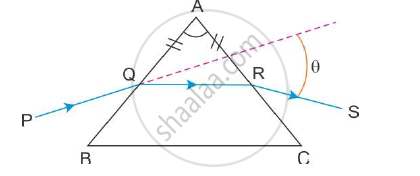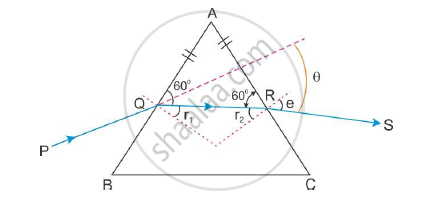Share

# A Ray PQ Incident on the Refracting Face BA is Refracted in the Prism BAC as Shown in the Figure and Emerges from the Other Refracting Face AC as RS Such that AQ = AR. - CBSE (Science) Class 12 - Physics

#### Question

A ray PQ incident on the refracting face BA is refracted in the prism BAC as shown in the figure and emerges from the other refracting face AC as RS such that AQ = AR. If the angle of prism A = 60° and refractive index of material of prism is sqrt3 . Calculate angle θ.#### Solution

The angle of the prism is A = 60°. It is also given that AQ = AR. Therefore, the angles opposite to these two sides are also equal.∠AQR = ∠ARQ

Now, for a triangle

∠A + ∠AQR + ∠ARQ = 180°

∴ ∠AQR = ∠ARQ = 60°

∴ r1=r2 = 30°  (∠AQO = ∠ARO = 90°)

∴ r1 + r2 = 60°

When r1 and r2 are equal, we have i = e. Now, according to Snell’s law,

mu=sini/sinr_1

:.sini=musinr_1=sqrt3 sin30 =sqrt3/2

∴ i = 60°

Now, the angle of deviation θ is

θ = i + e - A

∴ θ = 60 + 60 -60

∴  θ = 60°

Is there an error in this question or solution?

#### Video TutorialsVIEW ALL 

Solution A Ray PQ Incident on the Refracting Face BA is Refracted in the Prism BAC as Shown in the Figure and Emerges from the Other Refracting Face AC as RS Such that AQ = AR. Concept: Dispersion by a Prism.
S